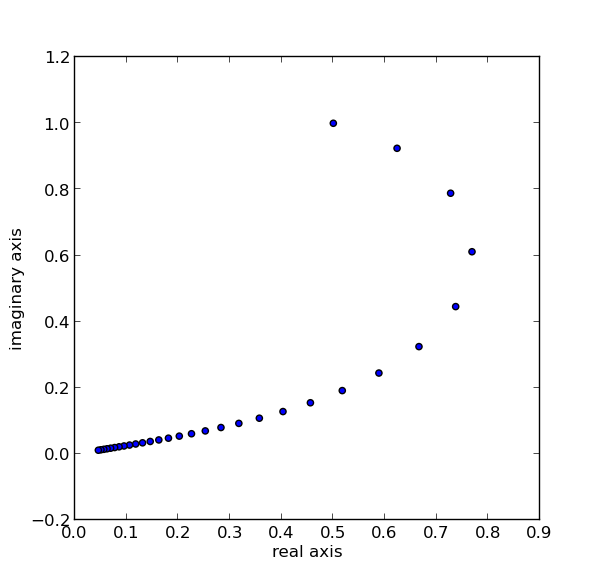# Iterating sines

Pick a starting point x0 and define x1 = f(x0), x2 = f(x1), x3 = f(x2) etc. For which functions and which starting points does the sequence converge? If the sequence converges, how quickly does it converge? In general these are hard questions, but this post will answer a couple special cases.

If f(x) = sin(0.9x) and x0 = 0.5 + i, here’s what the iterations look like:If the Taylor series for f(x) looks like a1x + a2x2 + a3x3 + … the iterates will converge if |a1| < 1 and your starting point is any complex number in a sufficiently small disk around the origin. The sequence might converge for starting points outside that disk, but there’s no guarantee.

The reason I chose sin(0.9x) for the example above rather than sin(x) is that the former has a1 = 0.9 and satisfies the theorem. The series for sin(x) has leading coefficient 1 and so the theorem doesn’t apply. For sin(x), the sequence of iterates does in fact converge starting at x0 = 0.5 + i but it diverges for other points, such as any purely imaginary starting point.

How fast do the iterates of f(x) converge if |a1| < 1? There is some constant b with |a1| < b < 1 such that | xn | < bn |x0 |. In other words, the sequence converges geometrically.

If we look at iterates of sin(x), the sequence converges for any starting value on the real line. The convergence is slower, on the order of 1/√n. And for points off the real line, the sequence may or may not converge.

Source: Asymptotic Methods in Analysis (Dover)

Update: See Mike Croucher’s blog post for a plot of the starting points where the sine iterates converge, repleat with Mathematica code.## 2 thoughts on “Iterating sines”

1. Among those who study complex dynamics (which subject includes the well-known Mandelbrot set and Julia sets of polynomials), this falls in the class of “transcendental dynamics”. See, for instance, “Dynamical properties of some classes of entire functions” by Eremenko and Lyubich and “Complex exponential dynamics” by Devaney. It’s not quite my field, so I’m not as familiar with the literature, but I did also find “A study of the dynamics of λsin(z)” by Domínguez and Sienra, which illustrates the Fatou sets for various values of λ.

2. victor

Would be nice if you also show the code for making those plots. Thanks!